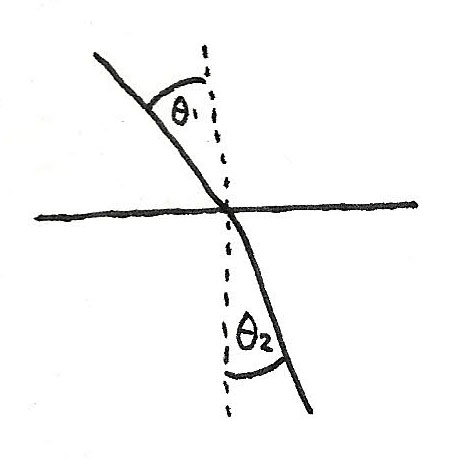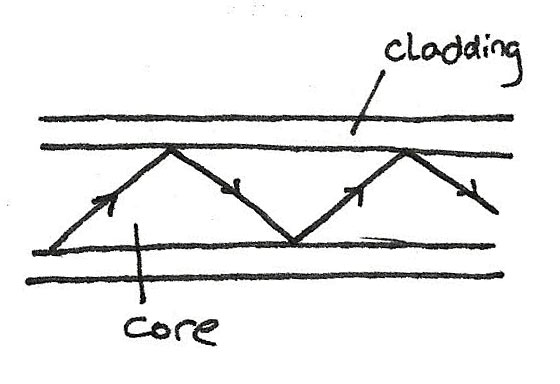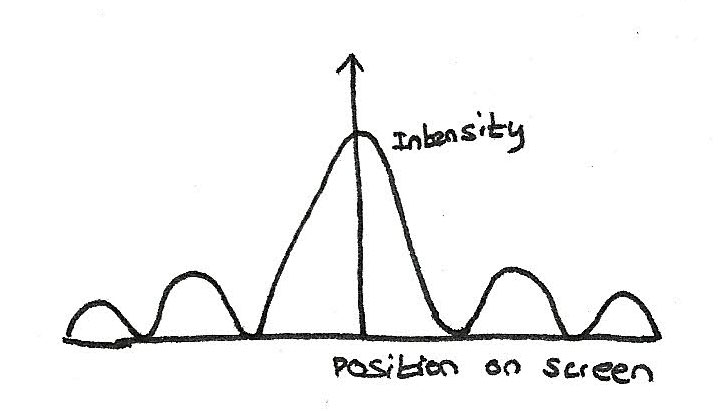# Optics

## Refraction of Light

Refraction is the change of direction of light passes through the boundary between two media. At a boundary between two substances, the light ray bends:

• Towards the normal if it passes into a more refractive substance.
• Away from the normal if it passes into a less refractive substance.

The refractive index, which is the ratio of the velocity of light in a medium compared to in a vacuum can be determined by measuring the angle of incidence and refraction:Then using Snell's Law:

$$n = \frac {\sin i}{\sin r}$$

where $n$ is the refractive index, $i$ is the angle of incidence and $r$ is the refractive index.

Refraction occurs due to the difference of the speed of light in each substance. When refraction occurs, the frequency doesn't change, although the wavelength and speed of the wave changes.

### Refraction Between Two Substances

When light passes from one substance to another substance, the speed of light in each substance can be calculated by using:

$$\frac {\sin i}{\sin r} = \frac {c_{1}}{c_{2}}$$By rearranging, multiplying both sides by the speed of light in a vacuum and substituting in the refractive indexes gives:

$$n_{1}{\sin i} = n_{2}{\sin r}$$

### White Light Spectrum

By using a prism, white light can be dispersed into the colours of the prism, which uses the principle of refraction. As each colour of the spectrum has a different wavelength, each colour refracts by a different amount. The shorter the wavelength, the greater the amount of refraction.

## Total Internal Reflection

When the angle of incidence reaches a certain angle, the light refracts along the boundary, before reflecting as the angle is increased. This is known as the critical angle. Total internal reflection can only occur if:

• The incident substance has a greater refractive index than the other substance.
• The angle of incidence exceeds the critical angle.

The critical angle $i_{c}$, can be calculated by using the following equation:

$$\sin i_{c} = \frac {n_{2}}{n_{1}}$$

This principle is what is used in optical fibres, to transmit information. The fibres are lined with cladding to prevent crossover of signals. In addition, the core is very narrow in order to prevent multi path dispersion, where due to the reflected light taking longer than the direct wave, the signal is received twice.## Double Slit Interference

To observe interference and the wave properties of light, two closely spaced parallel slits can be illuminated by a single light source. The two slits acts as coherent sources, which means they both emit light of the same frequency with the same phase difference. When this occurs, interference occurs and alternative light and dark fringes known as Young's fringes are formed.

The fringes are formed due to the interference of light:

• Where a bright fringe is formed, the constructive interference occurs.
• Where a dark fringe is formed, destructive interference occurs. This means that the light arrives $\pi$ radians out of phase.

The fringe separation can be calculated by using the equation:
$$w = \frac{\lambda D}{s}$$

Where $w$ is the fringe separation, $\lambda$ is the wavelength, $D$ is the distance from the slits to the screen, and $s$ is the spacing between slits.

### White Light fringes

In the double slit experiment, the fringe separation depends on the wavelength of the light used. This means that when white light is used, each colour component produces it's own pattern.

• The central fringe is white as every colour contributes to the centre.
• The inner fringes are tinged with blue on the inside and red on the outside. This is because red light spreads out more than blue.
• The outer fringes merge into a background of white light becoming fainter with increasing distance.

### Light Sources

Light from a filament light or from the sun are composed of the colours of the spectrum which without a filter, produce a spectrum of fringes.

Laser light is a monochromatic light source which can be used as a coherent source of light unlike a filament bulb. The issue with laser lights is that it can be dangerous if shined towards the eyes.

## Diffraction

When light passes through a gap, diffraction occurs where the wave spreads out. Diffracted waves spread out more if:

• The gap is made narrower
• The wavelength is made larger

Diffraction of light can be shown by shining a parallel beam of light at a single slit. The diffracted light produces a pattern of fringes on a white screen. The graph below shows the intensity of the light compared to the position on the screen.• The central fringe is twice as wide as the outer fringes.
• The peak intensity of each fringe decreases with distance from the centre.

### Observations of single slit diffraction

If the single slit pattern is observed:

• By using different sources of monochromatic light, the greater the wavelength, the wider the fringes.
• Making the slit narrower makes the fringes wider.

## Diffraction Grating

A diffraction grating consists of a plate with many closely spaced parallel slits. When a monochromatic light is shined through the grating, the light only travels in certain directions only as:

• The light passing through each slit is diffracted
• The diffracted light waves from adjacent slits reinforce in certain directions only.

The central, zero order beam is at the same direction as the incident beam. The other beams are of increasing order. The angle of diffraction between each transmitted beam and the central beam increases if:

• Light of a longer wavelength is used (eg by replacing blue filter with a red filter)
• A grating with closer slits is used.

An equation concerning the order the beams is:
$$d\sin \theta = n\lambda$$

Where $d$ is the spacing between adjacent slits, $sin \theta$ is the sine of the angle of diffraction, $n$ is the order of the beam and $\lambda$ is the wavelength of the wave.

• The number of slits per metre on the grating is:
$$N = \frac {1}{d}$$
where $d$ is the grating spacing.
• For a given order and wavelength, the smaller the value of $d$, the greater the angle of diffraction.
• The maximum number of orders is given by the value of $\frac {d}{\lambda}$ rounded down to the nearest whole number.